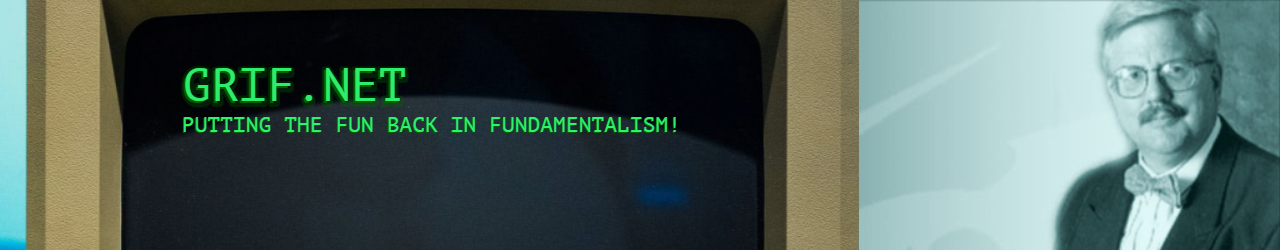# 04/05/17 Grif.Net – Increasing your Vocabulary

## 04/05/17 Grif.Net – Increasing your Vocabulary

Even native =
English speakers are constantly learning new words that they never knew =
existed, even though many have been around for hundreds of years. Just =
minefield that is English. Here are a few =E2=80=98new=E2=80=99 OLD =
words to use on your friends (or enemies) today

1. KERFLUFFLE =
(noun) 1800 =
from Scottish Gaelic for making a fuss or =
bother.

=E2=80=9CWhat=E2=
=80=99s all the shouting for? Why are you making such a =
kerfuffle?=E2=80=9D

2. =
HULLABALLOO
is the loud =
noises and shouting that people make when they=E2=80=99re angry. From =
1750 in England.

=E2=80=9CDid =
you hear all that hullaballoo in the office =
today?=E2=80=9D

3. CACOPHONY =
is a
nother noun =
related to noise, a mixture of horrible sounds. From the Greek =
kacos (bad) and phone (sound). It entered English in the =
mid 1600=E2=80=99s.

=E2=80=9CImagin=
e birds screeching, alarm bells ringing and babies screaming=E2=80=A6and =
you=E2=80=99ve got yourself a =
cacophony!=E2=80=9D

4. RAGAMUFFIN =
(noun) comes =
from the English that was used during the Middle Ages. From rag =
(
dirty piece of old cloth). It make sense to know that a =
ragamuffin is a person who wears dirty and scruffy clothes =
=E2=80=93 clothes that are just like rags! It=E2=80=99s usually used for =
children.

=E2=80=9CI =
send my children to school dressed smartly, and they come home like =
little ragamuffins!=E2=80=9D

5. =
WHIPPERSNAPPER has n
othing to do =
with whips or snaps. This noun from the 1600=E2=80=99s mixes a term for =
lazy person with no ambitions and juvenile delinquents always in =
trouble. Today it means overconfident know-it-all kids (who can program =
my iPhone) who question and correct their =
parents.

=E2=80=9CAnd =
yes, he really is a little =
whippersnapper!=E2=80=9D

6. =
GOBBLEDYGOOK.=C2=A0 What
sound does a =
turkey make? Does it sound something like =E2=80=9Cgobble, gobble, =
gobble=E2=80=9D? Created from the meaningless sound that turkeys make, =
this noun was created in the 1940=E2=80=99s to mean words that are =
nonsense or have no meaning.

=E2=80=9CThe =
boss was talking a load of gobbledygook in that meeting. I have no idea =
what he wants!=E2=80=9D

7. GIBBERISH. =
If
someone is =
talking gobbledygook they=E2=80=99ll also be talking =
gibberish! This old noun (pre-Shakespeare) came to English from =
someone in London listening to a speech in =
Spanish.

More of this =
gibberish tomorrow
😊 =

~~

Dr Bob Griffin =

bob@grif.net =
www.grif.net

"Jesus =
Knows Me, This I Love!"